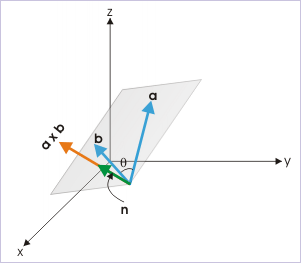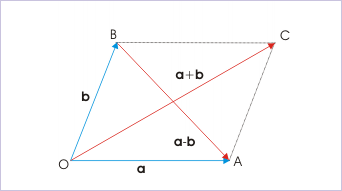1.10 Vector product (application)

 Page 1 / 1
Solving problems is an essential part of the understanding process.

Questions and their answers are presented here in the module text format as if it were an extension of the treatment of the topic. The idea is to provide a verbose explanation, detailing the application of theory. Solution presented is, therefore, treated as the part of the understanding process – not merely a Q/A session. The emphasis is to enforce ideas and concepts, which can not be completely absorbed unless they are put to real time situation.

Representative problems and their solutions

We discuss problems, which highlight certain aspects of the vector product. For this reason, questions are categorized in terms of the characterizing features of the subject matter :

• Condition of parallel vectors
• Unit vector of cross product
• Nature of vector product
• Evaluation of vector product
• Area of parallelogram

Condition of parallel vectors

Problem : Determine whether vectors 2 i j + 2 k and 3 i – 3 j + 6 k are parallel to each other?

Solution : If the two vectors are parallel, then ratios of corresponding components of vectors in three coordinate directions are equal. Here,

$\begin{array}{l}\frac{{a}_{x}}{{b}_{x}}=\frac{2}{3}\\ \frac{{a}_{y}}{{b}_{y}}=\frac{1}{3}\\ \frac{{a}_{z}}{{b}_{z}}=\frac{1}{3}\end{array}$

The ratios are, therefore, not equal. Hence, given vectors are not parallel to each other.

Unit vector of cross product

Problem : Find unit vector in the direction perpendicular to vectors i + j – 2 k and 2 i j + 3 k .

Solution : We know that cross product of two vectors is perpendicular to each of vectors. Thus, unit vector in the direction of cross product is perpendicular to the given vectors. Now, unit vector of cross product is given by :Unit vector in the direction of vector product.

$\begin{array}{l}\mathbf{n}=\frac{\mathbf{a}×\mathbf{b}}{|\mathbf{a}×\mathbf{b}|}\end{array}$

Here,

$\mathbf{a}×\mathbf{b}=|\begin{array}{ccc}\mathbf{i}& \mathbf{j}& \mathbf{k}\\ 1& 1& -2\\ 2& -1& 3\end{array}|$

$\begin{array}{l}⇒\mathbf{a}×\mathbf{b}=\left\{1\phantom{\rule{2pt}{0ex}}x\phantom{\rule{2pt}{0ex}}3-\left(-2\phantom{\rule{2pt}{0ex}}x\phantom{\rule{2pt}{0ex}}-1\right)\right\}\mathbf{i}+\left\{\left(2\phantom{\rule{2pt}{0ex}}x\phantom{\rule{2pt}{0ex}}-2\right)-1\phantom{\rule{2pt}{0ex}}x\phantom{\rule{2pt}{0ex}}3\right\}\mathbf{j}+\left\{\left(1\phantom{\rule{2pt}{0ex}}x\phantom{\rule{2pt}{0ex}}-1\right)-1\phantom{\rule{2pt}{0ex}}x\phantom{\rule{2pt}{0ex}}2\right\}\mathbf{k}\\ ⇒\mathbf{a}×\mathbf{b}=\mathbf{i}-7\mathbf{j}-3\mathbf{k}\end{array}$

$\begin{array}{l}⇒|\mathbf{a}×\mathbf{b}|=\surd \left\{{1}^{2}+{\left(-7\right)}^{2}+{\left(-3\right)}^{2}\right\}\end{array}$

$\begin{array}{l}⇒\mathbf{n}=\frac{1}{\surd \left(59\right)}\phantom{\rule{2pt}{0ex}}x\phantom{\rule{2pt}{0ex}}\left(\mathbf{i}-7\mathbf{j}-3\mathbf{k}\right)\end{array}$

Nature of vector product

Problem : Verify vector equality B = C , if AxB = AxC .

Solution : Let ${\theta }_{1}$ and ${\theta }_{2}$ be the angles for first and second pairs of cross products. Then,

$\begin{array}{l}\mathbf{A}×\mathbf{B}=\mathbf{A}×\mathbf{C}\\ ⇒\mathrm{AB}\mathrm{sin}{\theta }_{1}{\mathbf{n}}_{1}=\mathrm{AC}\mathrm{sin}{\theta }_{2}{\mathbf{n}}_{2}\\ ⇒B\mathrm{sin}{\theta }_{1}{\mathbf{n}}_{1}=C\mathrm{sin}{\theta }_{2}{\mathbf{n}}_{2}\end{array}$

It is clear that B = C is true only when $\mathrm{sin}{\theta }_{1}{\mathbf{n}}_{1}=\mathrm{sin}{\theta }_{2}{\mathbf{n}}_{2}$ . It is always possible that the angles involved or the directions of cross products are different. Thus, we can conclude that B need not be equal to C .

Evaluation of vector product

Problem : If a.b = | axb | for unit vectors a and b , then find the angle between unit vectors.

Solution : According to question,

$\begin{array}{l}\mathbf{a}\mathbf{.}\mathbf{b}=|\mathbf{a}×\mathbf{b}|\\ ⇒\mathrm{ab}\mathrm{cos}\theta =\mathrm{ab}\mathrm{sin}\theta \\ ⇒\mathrm{tan}\theta =1\phantom{\rule{2pt}{0ex}}x\phantom{\rule{2pt}{0ex}}1\phantom{\rule{2pt}{0ex}}x\phantom{\rule{2pt}{0ex}}\mathrm{tan}45°\\ ⇒\theta =45°\end{array}$

Problem : Prove that :

$\begin{array}{l}{|\mathbf{a}\mathbf{.}\mathbf{b}|}^{2}-{|\mathbf{a}×\mathbf{b}|}^{2}={a}^{2}\phantom{\rule{2pt}{0ex}}x\phantom{\rule{2pt}{0ex}}{b}^{2}\phantom{\rule{2pt}{0ex}}x\phantom{\rule{2pt}{0ex}}\mathrm{cos}\mathrm{2\theta }\end{array}$

Solution : Expanding LHS, we have :

$\begin{array}{l}{|\mathbf{a}\mathbf{.}\mathbf{b}|}^{2}-{|\mathbf{a}×\mathbf{b}|}^{2}={\left(\mathrm{ab}\mathrm{cos}\theta \right)}^{2}-{\left(\mathrm{ab}\mathrm{sin}\theta \right)}^{2}={a}^{2}{b}^{2}\left({\mathrm{cos}}^{2}\theta -{\mathrm{sin}}^{2}\theta \right)\\ ⇒{|\mathbf{a}\mathbf{.}\mathbf{b}|}^{2}-{|\mathbf{a}×\mathbf{b}|}^{2}=={a}^{2}{b}^{2}\mathrm{cos}2\theta \end{array}$

Area of parallelogram

Problem : The diagonals of a parallelogram are represented by vectors 3 i + j + k and i - j - k . Find the area of parallelogram.

Solution : The area of parallelogram whose sides are formed by vectors a and b , is given by :

$\begin{array}{l}\mathrm{Area}=|\mathbf{a}×\mathbf{b}|\end{array}$

However, we are given in question vectors representing diagonals – not the sides. But, we know that the diagonals are sum and difference of vectors representing sides of a parallelogram. It means that :The vectors along diagonals are sum and difference of two vectors representing the sides.

$\begin{array}{l}\mathbf{a}+\mathbf{b}=3\mathbf{i}+\mathbf{j}+\mathbf{k}\end{array}$

and

$\begin{array}{l}\mathbf{a}-\mathbf{b}=\mathbf{i}-\mathbf{j}-\mathbf{k}\end{array}$

Now the vector product of vectors representing diagonals is :

$\begin{array}{l}\left(\mathbf{a}+\mathbf{b}\right)×\left(\mathbf{a}-\mathbf{b}\right)=\mathbf{a}×\mathbf{a}-\mathbf{a}×\mathbf{b}+\mathbf{b}×\mathbf{a}+\mathbf{b}×\left(-\mathbf{b}\right)=-\mathbf{a}×\mathbf{b}+\mathbf{b}×\mathbf{a}\end{array}$

Using anti-commutative property of vector product,

$\begin{array}{l}\left(\mathbf{a}+\mathbf{b}\right)×\left(\mathbf{a}-\mathbf{b}\right)=-2\mathbf{a}×\mathbf{b}\end{array}$

Thus,

$\begin{array}{l}\mathbf{a}×\mathbf{b}=-\frac{1}{2}\phantom{\rule{2pt}{0ex}}x\phantom{\rule{2pt}{0ex}}\left(\mathbf{a}+\mathbf{b}\right)×\left(\mathbf{a}-\mathbf{b}\right)\end{array}$

$\mathbf{a}×\mathbf{b}=-\frac{1}{2}|\begin{array}{ccc}\mathbf{i}& \mathbf{j}& \mathbf{k}\\ 3& 1& 1\\ 1& -1& -1\end{array}|$

$\begin{array}{l}\mathbf{a}×\mathbf{b}=-\frac{1}{2}\phantom{\rule{2pt}{0ex}}x\phantom{\rule{2pt}{0ex}}\left\{\left(1\phantom{\rule{2pt}{0ex}}x\phantom{\rule{2pt}{0ex}}-1-1\phantom{\rule{2pt}{0ex}}x\phantom{\rule{2pt}{0ex}}-1\right)\mathbf{i}+\left(1\phantom{\rule{2pt}{0ex}}x\phantom{\rule{2pt}{0ex}}1-3\phantom{\rule{2pt}{0ex}}x\phantom{\rule{2pt}{0ex}}-1\right)\mathbf{j}+\left(3\phantom{\rule{2pt}{0ex}}x\phantom{\rule{2pt}{0ex}}-1-1\phantom{\rule{2pt}{0ex}}x\phantom{\rule{2pt}{0ex}}1\right)\mathbf{k}\right\}\\ \mathbf{a}×\mathbf{b}=-\frac{1}{2}\phantom{\rule{2pt}{0ex}}x\phantom{\rule{2pt}{0ex}}\left(4\mathbf{j}-4\mathbf{k}\right)\\ \mathbf{a}×\mathbf{b}=-2\mathbf{j}+2\mathbf{k}\end{array}$

The volume of the parallelogram is :

$\begin{array}{l}|\mathbf{a}×\mathbf{b}|=\surd \left\{{\left(-2\right)}^{2}+{2}^{2}\right\}=2\surd 2\phantom{\rule{2pt}{0ex}}\mathrm{units}\end{array}$

what's lamin's theorems and it's mathematics representative
if the wavelength is double,what is the frequency of the wave
What are the system of units
A stone propelled from a catapult with a speed of 50ms-1 attains a height of 100m. Calculate the time of flight, calculate the angle of projection, calculate the range attained
58asagravitasnal firce
Amar
water boil at 100 and why
what is upper limit of speed
what temperature is 0 k
Riya
0k is the lower limit of the themordynamic scale which is equalt to -273 In celcius scale
Mustapha
How MKS system is the subset of SI system?
which colour has the shortest wavelength in the white light spectrum
how do we add
if x=a-b, a=5.8cm b=3.22 cm find percentage error in x
x=5.8-3.22 x=2.58
what is the definition of resolution of forces
what is energy?
Ability of doing work is called energy energy neither be create nor destryoed but change in one form to an other form
Abdul
motion
Mustapha
highlights of atomic physics
Benjamin
can anyone tell who founded equations of motion !?
n=a+b/T² find the linear express
أوك
عباس
QuiklyyyByByBy